Latest Banking jobs   »   Reasoning Ability Quiz For Bank Mains...

# Reasoning Ability Quiz For Bank Mains Exams 2021- 2nd February

Directions (1-5): Study the following information carefully and answer the questions given below:
Seven boxes A, B, C, D, E, F, G are kept one above the other containing different number of chocolates. Not more than three boxes are kept below A. Two boxes are kept between A and the box containing 41 chocolates, which is kept above Box A. D contains thrice the number of chocolates than box B. Box C contains 50 number of chocolates. The number of chocolates in box G is a cube of a number. Only one box is kept between box containing 41 chocolates and the box which contains 39 chocolates. Only one box is between the box containing 50 chocolates and the one which contains 39 chocolates. Two boxes are between the box containing 50 chocolates and the one which contains 64 chocolates. The number of boxes between C and D is same as the number of boxes between C and the box which contains 13 chocolates. Box F contains 80 chocolates. One box contains 83 chocolates.

Q1. How many box/boxes is/are kept between Box D and Box B?
(a) one
(b) two
(c) three
(d) more than three
(e) none

Q2. How many chocolates are kept in box E?
(a) 50
(b) 13
(c) 83
(d) 41
(e) none of these

Q3. Which among the following boxes contains the maximum and minimum number of chocolates respectively?
(a) G, E
(b) B, D
(c) B, A
(d) A, B
(e) none of these

Q4. Which of the following combination is not true?
(a) 50-C
(b) 13-B
(c) 41-E
(d) 64-G
(e) All are true

Q5. Which among the following boxes is kept immediately below box B?
(a) G
(b) C
(c) A
(d) No One
(e) none of these

Directions (6-10): Each of the following questions below consists of a question and two statements numbered I and II given below it. You have to decide whether the data provided in the statements are sufficient to answer the question. Read both the statements and give answer.

Q6. What does ‘ra’ mean in the code language?
I. ‘st qm ra’ means ‘Look at me’ and ‘qm ms st rt’ means ‘don’t look at him’ in that code language.
II. ‘ka tv ne ra’ means ‘take me and go’ and ‘vw wx si ra’ means ‘either me or you’ in that code language.
(a) if the data in statement I alone are sufficient to answer the question, while the data in statement II alone are not sufficient in answer the question.
(b) if the data in statement II alone are sufficient to answer the question, while the data in statement I alone are not sufficient to answer the question.
(c) if the data in either in statement I alone or in statement II alone are sufficient to answer the question.
(d) if the data in both the statements I and II together are not sufficient to answer the question.
(e) if the data in both the statements I and II are together necessary to answer the question.

Q7. How is Sarita related to Rajesh?
I. Soni, the cousin of Rajesh, is the niece of Sarita.
II. Swarna is sister of Sarita who is the wife of Om. Om is the father of Rajesh.
(a) if the data in statement I alone are sufficient to answer the question, while the data in statement II alone are not sufficient in answer the question.
(b) if the data in statement II alone are sufficient to answer the question, while the data in statement I alone are not sufficient to answer the question.
(c) if the data in either in statement I alone or in statement II alone are sufficient to answer the question.
(d) if the data in both the statements I and II together are not sufficient to answer the question.
(e) if the data in both the statements I and II are together necessary to answer the question.

Q8. What is M’s rank from the top in the class of 40?
I. The rank of S is 9th from the top and R’s rank is 20th from the bottom. M has a higher rank than R.
II. N, who is between M and Q, is 15th from the bottom.
(a) if the data in statement I alone are sufficient to answer the question, while the data in statement II alone are not sufficient to answer the question.
(b) if the data in statement II alone are sufficient to answer the question, while the data in statement I alone are not sufficient to answer the question.
(c) if the data in either in statement I alone or in statement II alone are sufficient to answer the question.
(d) if the data in both the statements I and II together are not sufficient to answer the question.
(e) if the data in both the statements I and II are together necessary to answer the question.

Q9. Point Y is in which direction with respect to point X?
I. Point A is east of point B and north of point X. Point Y is north-west of point A.
II. Point X is east of point B and north of point A. Point Y is north of point A.
(a) if the data in statement I alone are sufficient to answer the question, while the data in statement II alone are not sufficient in answer the question.
(b) if the data in statement II alone are sufficient to answer the question, while the data in statement I alone are not sufficient to answer the question.
(c) if the data in either in statement I alone or in statement II alone are sufficient to answer the question.
(d) if the data in both the statements I and II together are not sufficient to answer the question.
(e) if the data in both the statements I and II are together necessary to answer the question.

Q10. Who among A, B, C, D and E types fastest?
I. B types faster than E but he is not the fastest among them.
II. C types faster than D and E. C does not type as fast as A and B.
(a) if the data in statement I alone are sufficient to answer the question, while the data in statement II alone are not sufficient in answer the question.
(b) if the data in statement II alone are sufficient to answer the question, while the data in statement I alone are not sufficient to answer the question.
(c) if the data in either in statement I alone or in statement II alone are sufficient to answer the question.
(d) if the data in both the statements I and II together are not sufficient to answer the question.
(e) if the data in both the statements I and II are together necessary to answer the question.

Directions (11-12): Study the following information carefully and answer the given questions:
In a family there are eight members. A is mother of B, who is brother of C. C and Q are siblings. Y is daughter of P. Z is brother in law of B, who is son of D. A has only one son. There are only two married couples in the family. P is married to B.

Q11. How is P related with Z?
(a) Brother
(b) Daughter
(c) Nephew
(d) Niece
(e) None of these

Q12. How is C related with Y?
(a) Aunt
(b) Daughter in law
(c) Uncle
(d) Granddaughter
(e) none of these

Directions (13-15): These questions are based on the following information.
‘P@Q’ means ‘Q is the mother of P’
‘P\$Q’ means ‘Q is the husband of P’
‘P#Q’ means ‘Q is the sister of P’
‘P*Q’ means ‘Q is the son of P’.

Q13. If ‘A # B \$ C * D * E’ then how is E related with B?
(a) son
(b) daughter
(c) Grand daughter
(d) Grand son
(e) None of these

Q14. If ‘A * B @ C * D # E’ then how is A related with E?
(a) son
(b) daughter
(c) Father
(d) mother
(e) None of these

Q15. If ‘A#B\$C*D#E’ then how is B related with E?
(a) son
(b) daughter
(c) Father
(d) mother
(e) None of these

Practice More Questions of Reasoning for Competitive Exams:

###### Bank Mains Cracker Study Plan 2021 : Check Now

Solutions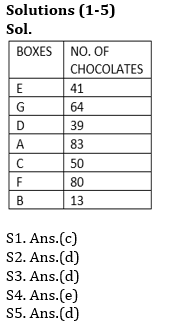S6. Ans.(c)
Sol. if the data in either in statement I alone or in statement II alone are sufficient to answer the question. ra=me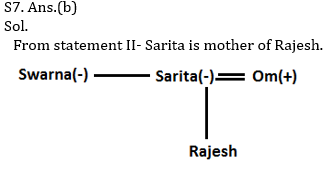S8. Ans.(d)

S9. Ans.(a)
Sol. if the data in statement I alone are sufficient to answer the question, while the data in statement II alone are not sufficient in answer the question.
From Statement I- Point Y is in north-west of point X.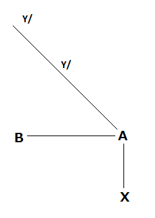S10. Ans.(e)
Sol. if the data in both the statements I and II are together necessary to answer the question.
After combining both statements I and II- A types fastest among them.
A>B>C>D/E>D/E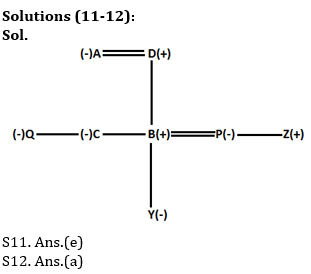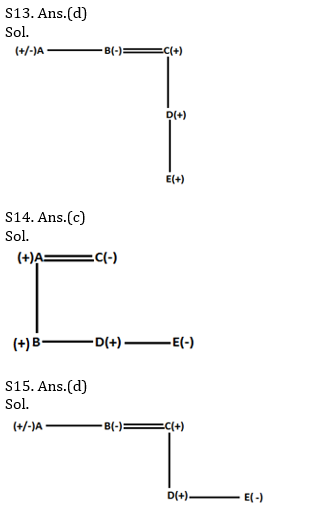×

Thank You, Your details have been submitted we will get back to you.Join India's largest learning destination

What You Will get ?

•Job Alerts
•Daily Quizzes
•Subject-Wise Quizzes
•Current Affairs
•Previous year question papers
•Doubt Solving session

ORJoin India's largest learning destination

What You Will get ?

•Job Alerts
•Daily Quizzes
•Subject-Wise Quizzes
•Current Affairs
•Previous year question papers
•Doubt Solving session

ORJoin India's largest learning destination

What You Will get ?

•Job Alerts
•Daily Quizzes
•Subject-Wise Quizzes
•Current Affairs
•Previous year question papers
•Doubt Solving session

Enter the email address associated with your account, and we'll email you an OTP to verify it's you.Join India's largest learning destination

What You Will get ?

•Job Alerts
•Daily Quizzes
•Subject-Wise Quizzes
•Current Affairs
•Previous year question papers
•Doubt Solving session

Enter OTP

Please enter the OTP sent to
/6

Did not recive OTP?

Resend in 60sJoin India's largest learning destination

What You Will get ?

•Job Alerts
•Daily Quizzes
•Subject-Wise Quizzes
•Current Affairs
•Previous year question papers
•Doubt Solving sessionJoin India's largest learning destination

What You Will get ?

•Job Alerts
•Daily Quizzes
•Subject-Wise Quizzes
•Current Affairs
•Previous year question papers
•Doubt Solving session

Almost there

+91Join India's largest learning destination

What You Will get ?

•Job Alerts
•Daily Quizzes
•Subject-Wise Quizzes
•Current Affairs
•Previous year question papers
•Doubt Solving session

Enter OTP

Please enter the OTP sent to Edit Number

Did not recive OTP?

Resend 60

By skipping this step you will not recieve any free content avalaible on adda247, also you will miss onto notification and job alerts

Are you sure you want to skip this step?

By skipping this step you will not recieve any free content avalaible on adda247, also you will miss onto notification and job alerts

Are you sure you want to skip this step?